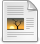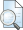# How to generate Pythagorean triples - 1

Shirali, Shailesh (2012) How to generate Pythagorean triples - 1. At Right Angles, 1 (1). pp. 29-32.Text - Published Version Download (892kB)

## Abstract

The relation a^2+b^2=c^2 is so familiar to us that we often quote it without saying what a, b, c represent! And this, no doubt, is because the Pythagorean theorem is so well known. We know that if a, b, c are the sides of a right angled triangle, with c as the hypotenuse, then a^2 +b^2=c^2. We also know, conversely, that if a, b, c are positive numbers which satisfy this relation, then one can construct a right angled triangle with legs a, b and hypotenuse c. Because of this association, we call a triple (a, b, c) of positive integers satisfying this relation a Pythagorean triple, PT for short. But such triples have additional properties of interest that have nothing to do with their geometric origins; they have number theoretic properties, and we will be studying some of them in this and some follow up articles.

Item Type: Articles in APF Magazines
Authors: Shirali, Shailesh
Document Language:
Language
English
Uncontrolled Keywords: Generate, Pythagorean,Triples, Number theoretic, Odd Squares
Subjects: Natural Sciences > Mathematics
Divisions: Azim Premji University > University Publications > At Right Angles
Full Text Status: Public
URI: http://publications.azimpremjiuniversity.edu.in/id/eprint/1577
Publisher URL:View Item